Java Games: Flashcards, matching, concentration, and word search.# Matching Polynomial Functions and Graphs

AB
f(x) = -2x +3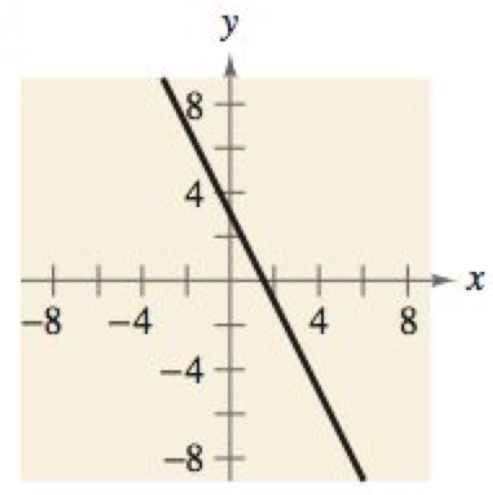f(x) = x^2 - 4x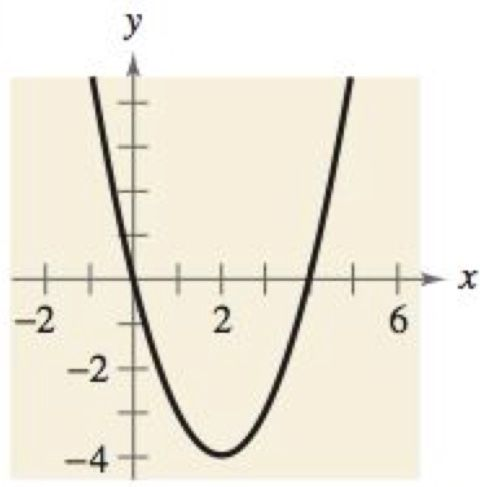f(x) = -2x^2 -5x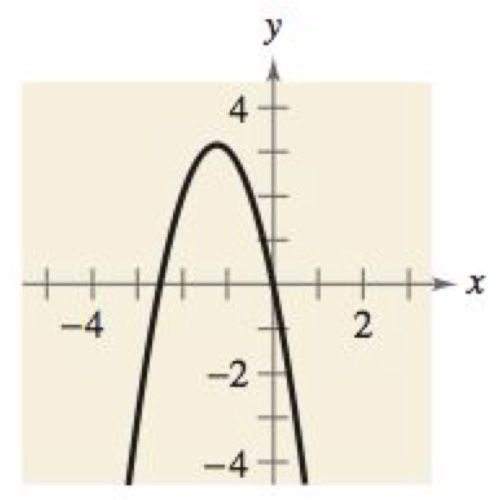f(x) = 2x^3 - 3x + 1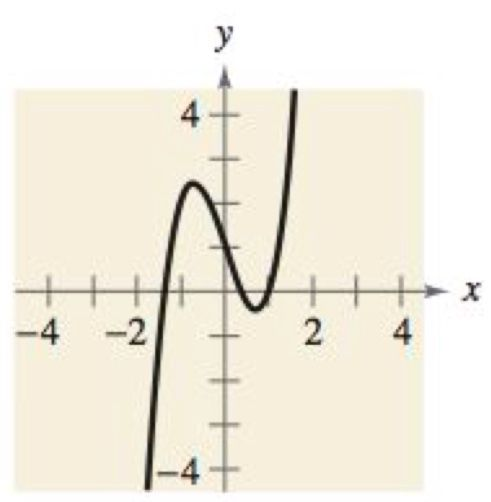f(x) = -(1/4)x^4 + 3x^2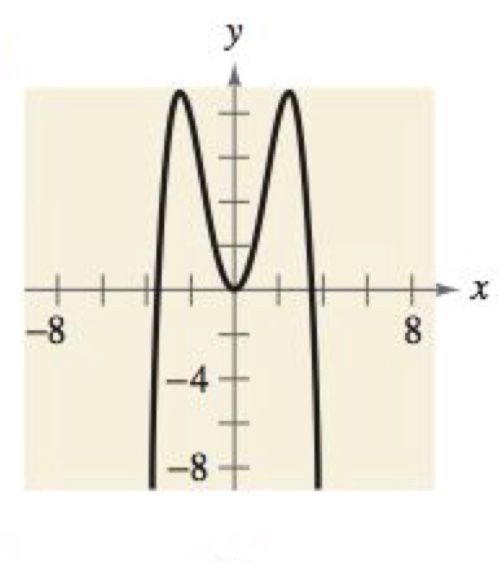f(x) = -(1/3)x^3 + x^2 - (4/3)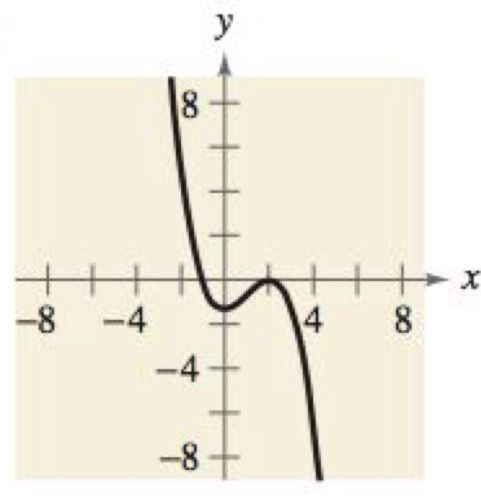f(x) = x^4 + 2x^3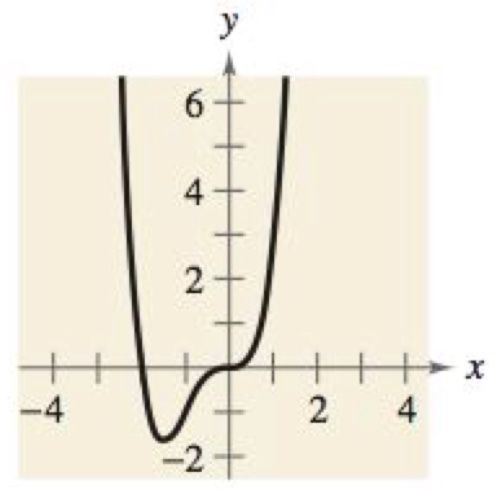f(x) = (1/5)x^5 - 2x^3 + (9/5)xMrs. Miller math teacher Susquenita High School Duncannon, PA

 This activity was created by a Quia Web subscriber. Learn more about QuiaCreate your own activities